Faster R-CNN is an object detecting network proposed in 2015, and achieved state-of-the-art accuracy on several object detection competitions.

# Introduction

## Summary

• Problem Statement
• Even though SPPnet and Fast R-CNN have reduced the running time of object detection networks, they have region proposal computation as a bottleneck.
• Research Objective
• To improve object detection networks in terms of speed and accuracy
• Solution Proposed: Faster R-CNN
• Faster R-CNN is a single network of combination of RPN and Fast R-CNN by sharing their convolutional features.
• Introduce a Region Proposal Network (RPN) that shares full-image convolutional features with the detection network to get cost-free region proposals.
• Contribution
• For the very deep VGG-16 model, proposed detection system has a frame rate of 5fps on a GPU.
• Achieved state-of-the-art object detection accuracy on PASCAL VOC 2007, 2012, and MS COCO datasets with only 300 proposals per image.
• In ILSVRC and COCO 2015 competitions, Faster R-CNN and RPN are the foundations of the 1st-place winning entries in several tracks.

## R-CNN Series

1) R-CNN: Rich feature hierarchies for accurate object detection and semantic segmentation (2013)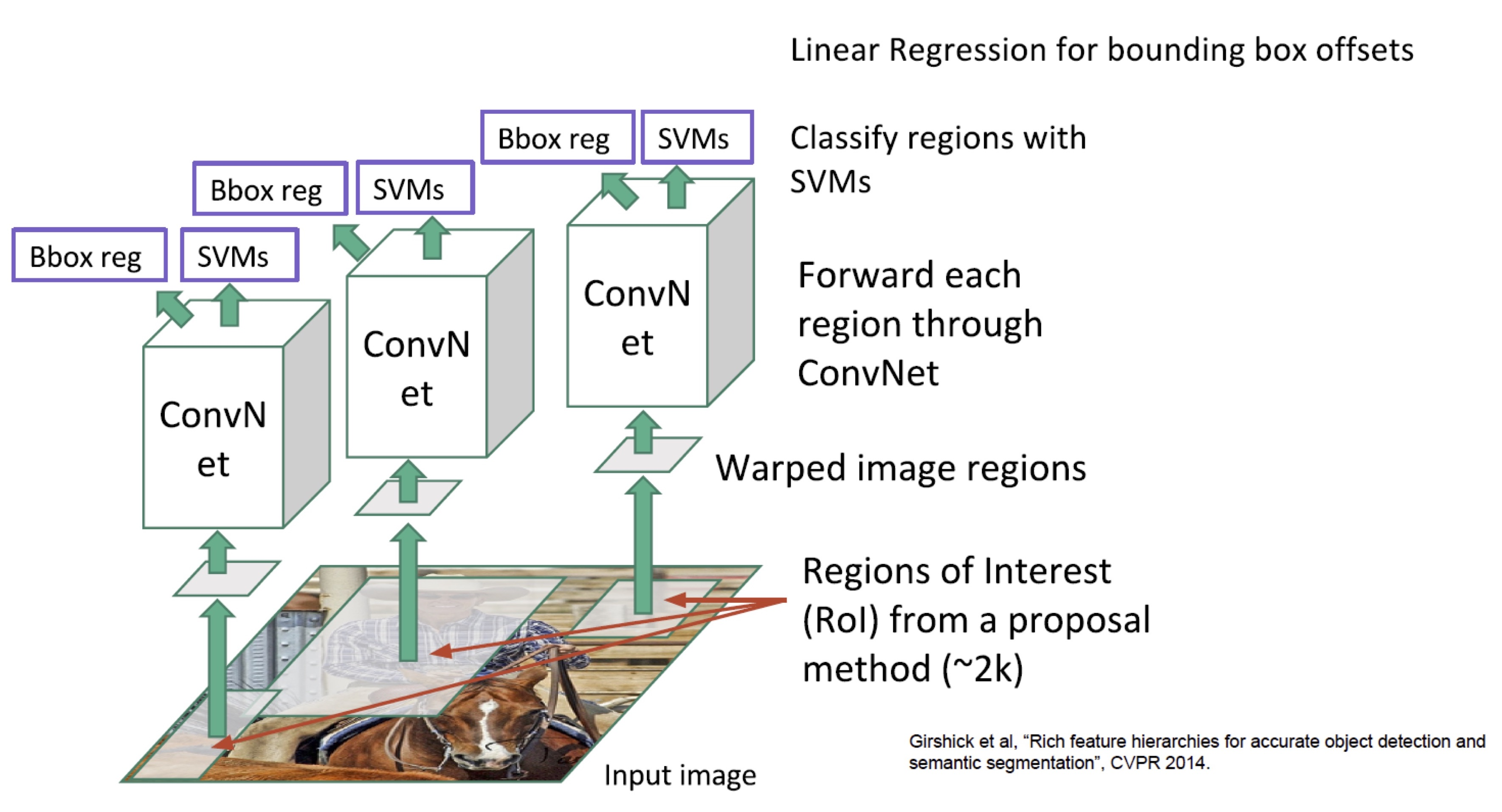• Region Proposals: Selective Search

• Training R-CNN
• Pre-train a CNN(AlexNet) for ImageNet classification dataset
• Fine-tune for object detection (softmax + log loss)
• Cache feature vectors to disk
• Train post hoc (parameters learned after CNN is fixed) linear SVM (hinge loss)
• Train post hoc linear bounding-box regressors (squared loss)
• Bounding-Box Regression
• Train a linear regression classifier that will output some correction factor
• Problem of R-CNN
• Slow at test-time: need to run full forward path of CNN for each region proposal
• Takes 13s/image on a GPU (K40) and 53s/image on a CPU while testing
• SVM and regressors are post-hoc: CNN features are not updated in response to SVMs and regressors
• Complex multistage training pipeline (84 hours using K40 GPU)
• Fine-tune network with softmax classifier (log loss)
• Train post-hoc linear SVMs (hinge loss)
• Train post-hoc bounding-box regressions (squared loss)

2) Fast R-CNN (2015)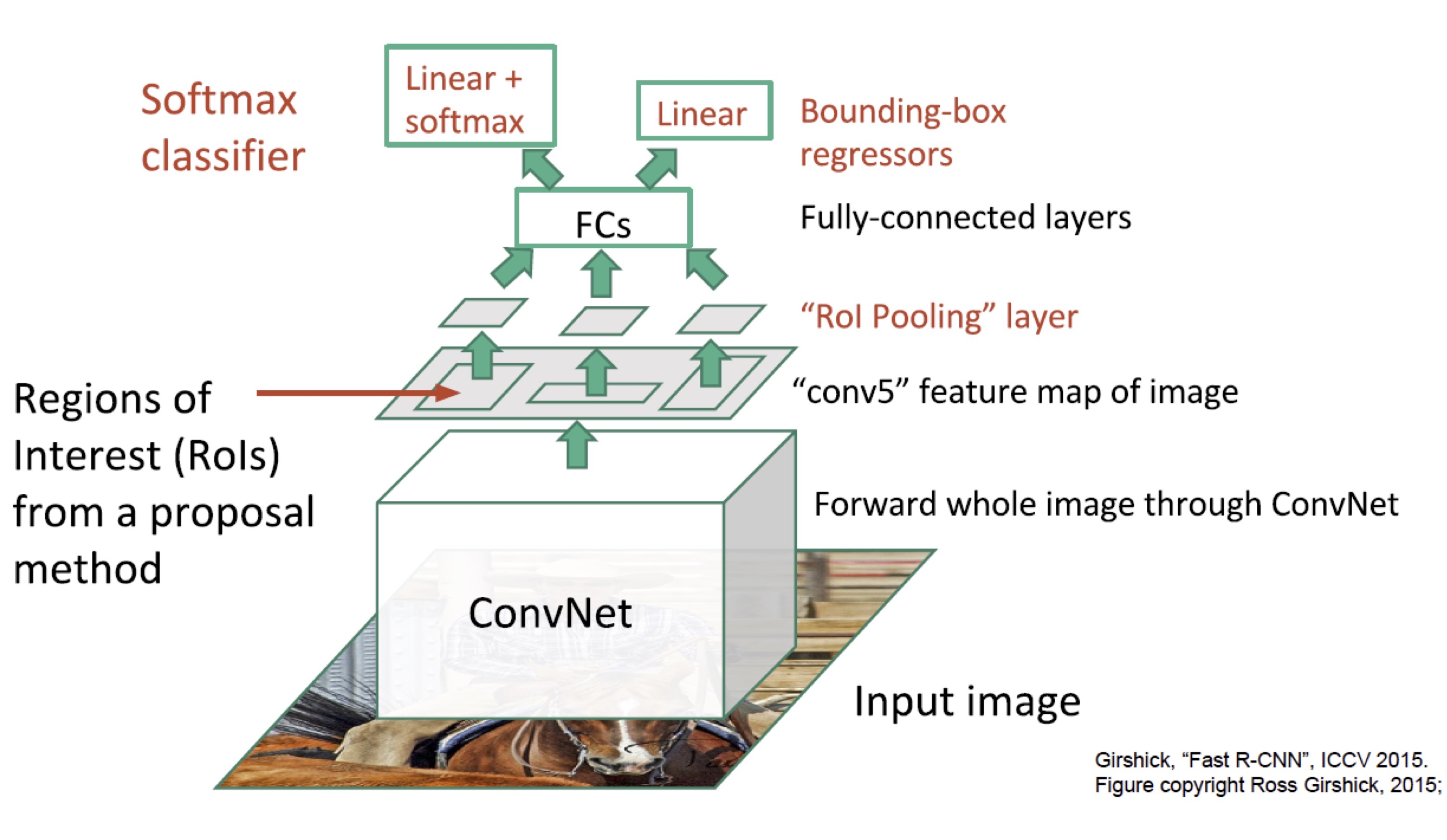• Fast R-CNN improved drawbacks of R-CNN and SPP-net
• Train the detector in a single stage, end-to-end without caching features or post hoc training steps
• Train all layers of the network
• RoI pooling
• It is a type of max-pooling with a pool size dependent on the input, so that the output always has the same size.
• Fully connected layer always expected the same input size.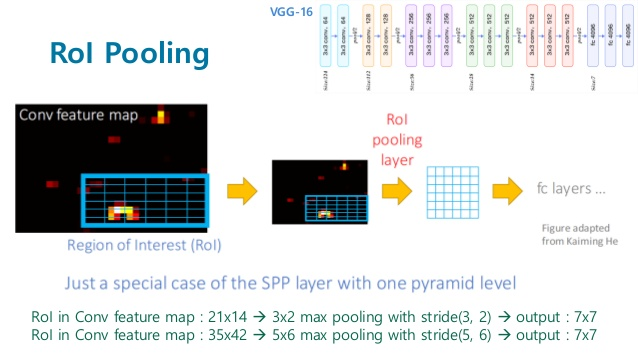• Problems of Fast R-CNN
• Still depends on external system to give the region proposals (selective search)
• It is computational bottleneck for test-time as the algorithm learns on CPU

3) Faster R-CNN: Towards Real-Time Object Detection with Region Proposal Networks (2015)

## Speed Comparison of Object Detectors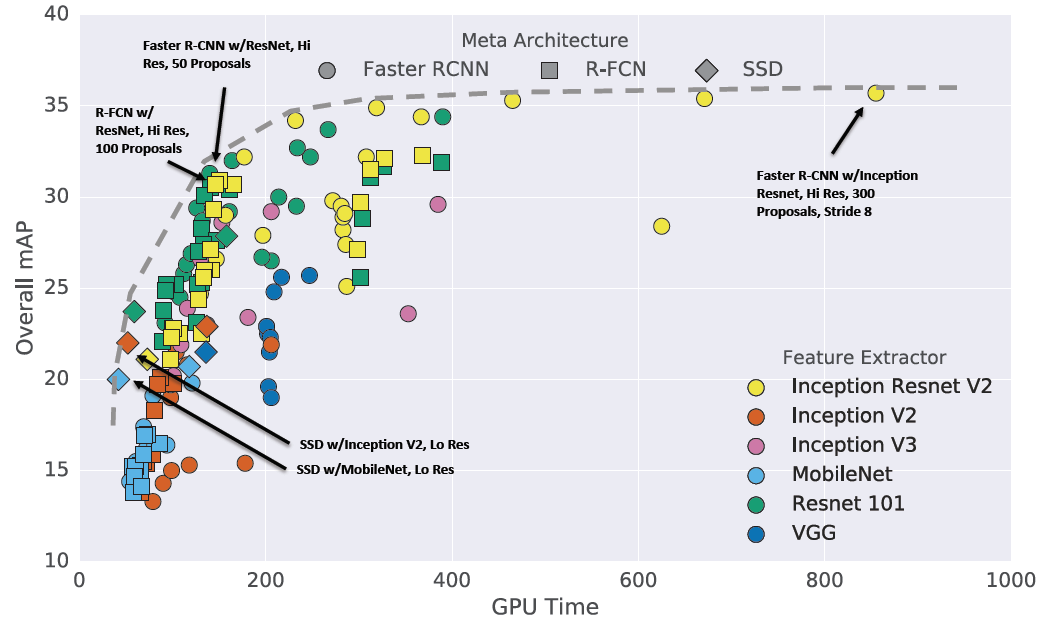• Generally R-FCN and SSD models are faster on average while Faster R-CNN models are more accurate.
• Faster R-CNN models can be faster if we limit the number of regions proposed.

# Faster R-CNN

• Faster R-CNN: RPN + Fast R-CNN
• Insert a Region Proposal Network (RPN) after the last convolutional layer using GPU
• RPN trained to produce region proposals directly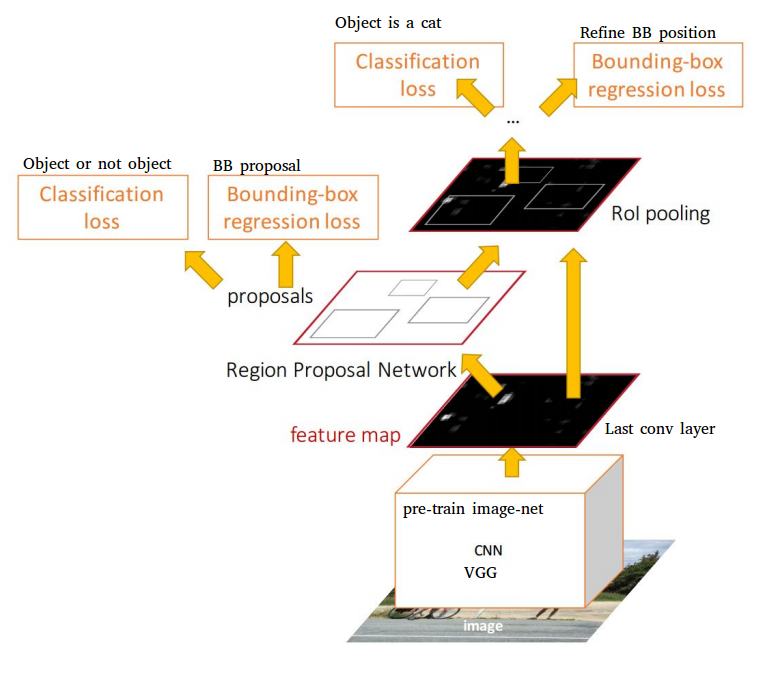## Region Proposal Network (RPN)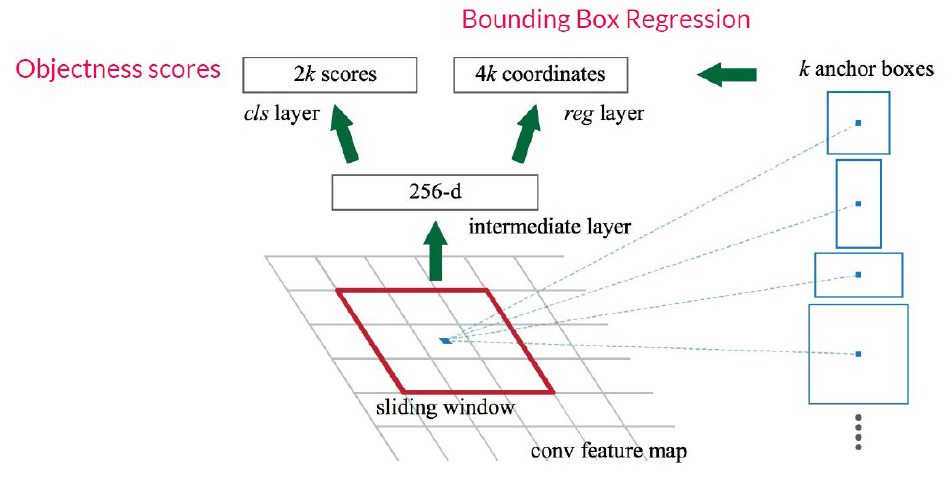• RPN
• Slide a small window on the feature map
• Build a small network for classifying object or not-object and regressing bounding-box locations
• Position of the sliding window provides localization information with reference to the image
• Box regression provides finer localization information with reference to this sliding window
• Use k anchor boxes at each location as translation invariant
• Regression gives offsets from anchor boxes
• Classification gives the probability that each anchor shows an object
• Anchors: pre-defined reference boxes
• Multiple anchors are used at each position
• Each anchor has its own prediction function
• Single-scale features, multi-scale predictions

## 4- Step Alternating Training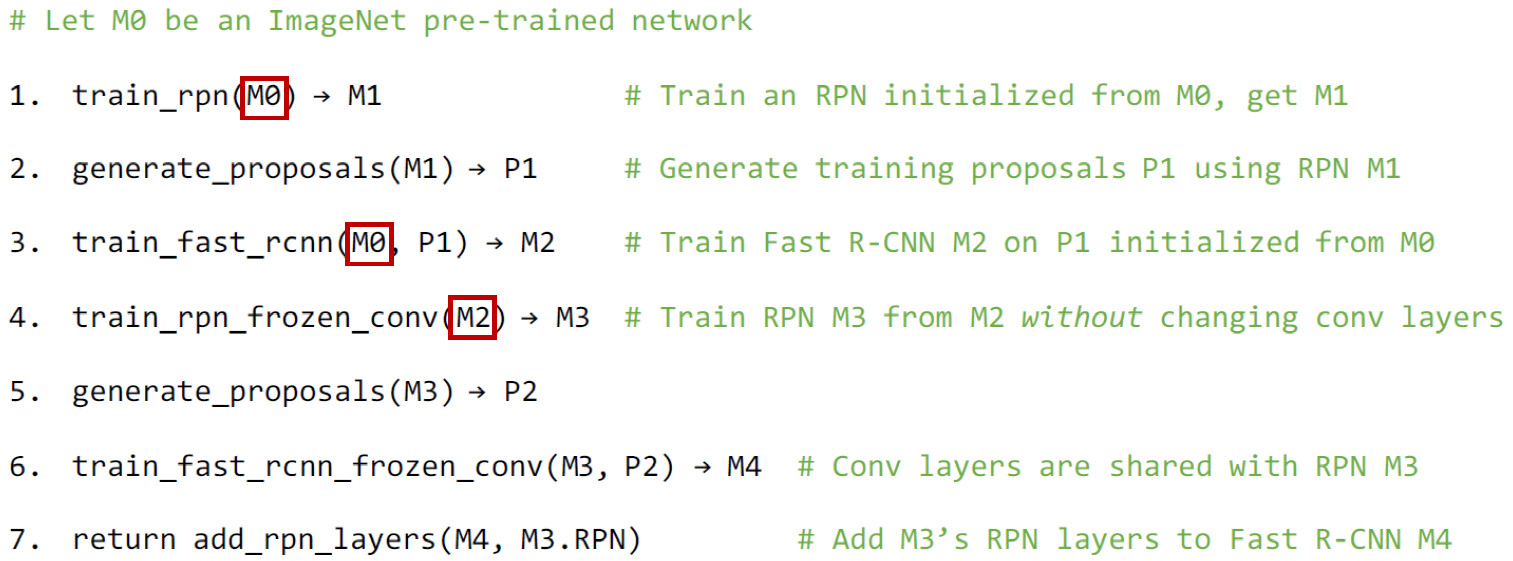# Experiments

• Speed Comparision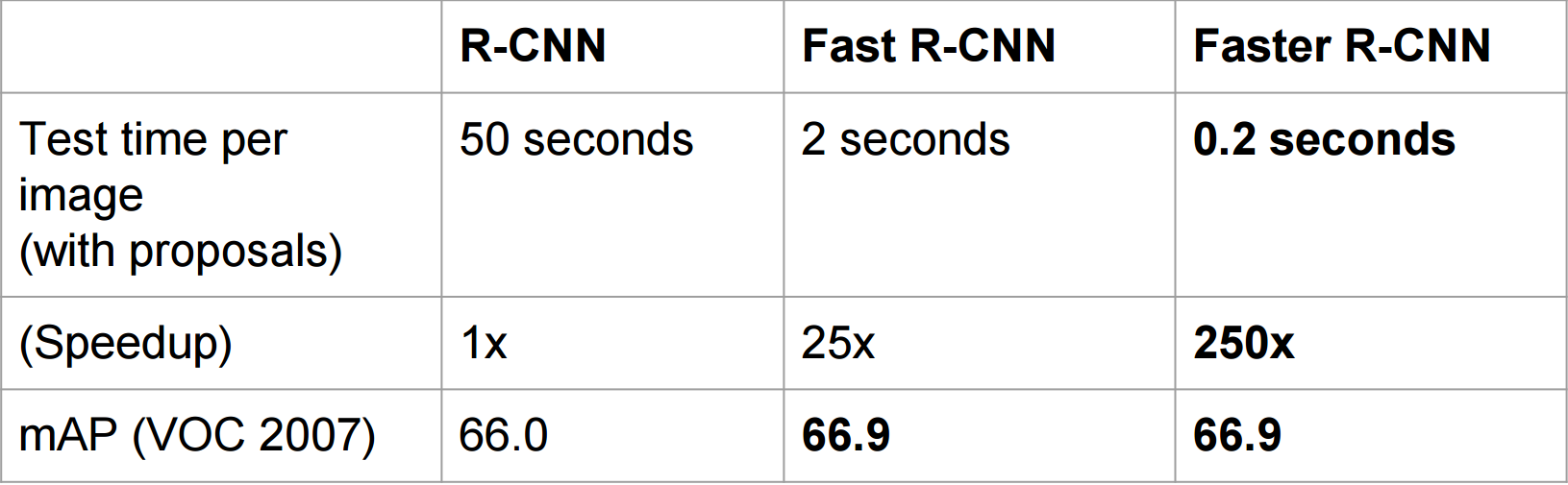• Detection results on PASCAL VOC 2007 test set

• Using RPN yields a much faster detection system than using either SS or EB because of shared convolutional computations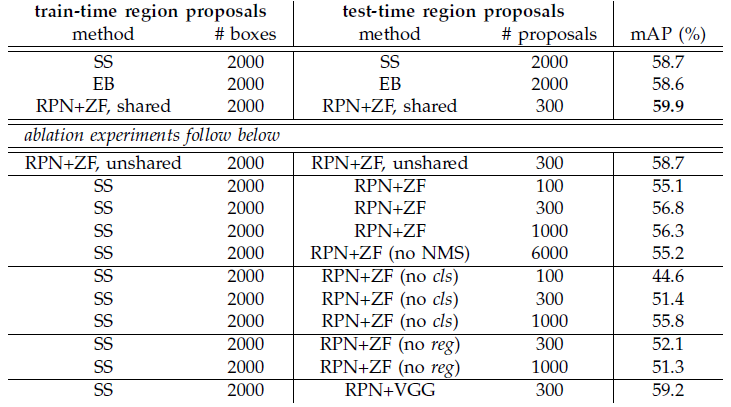• Timing (ms) on a K40 GPU, except SS proposal is evaluated in a CPU
• Using RPN gives a much faster running time of the entire object detection system.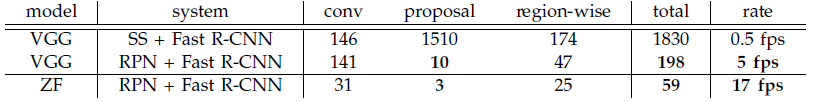• Problem of Faster R-CNN
• RoI pooling has quantization operations which can cause misalignments between the RoI and the extracted features
• Even though this would not impact classification, it can make a negative effect on predicting bounding box